# 2 Unit 1. Traditional Symmetric Ciphers.pdf

30 de May de 2023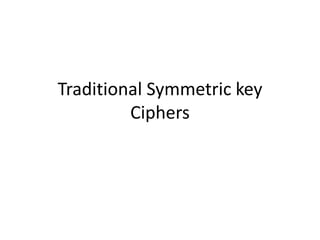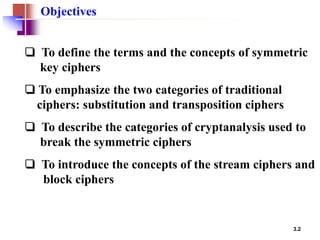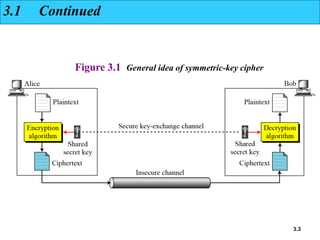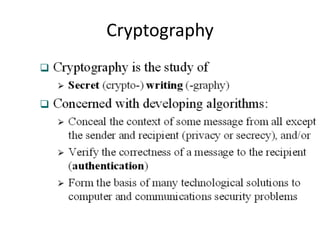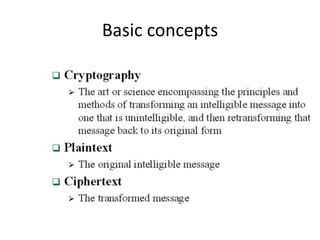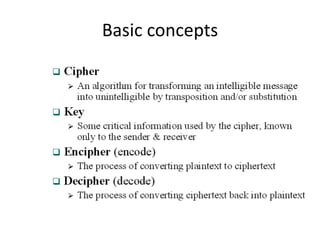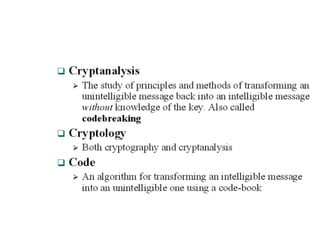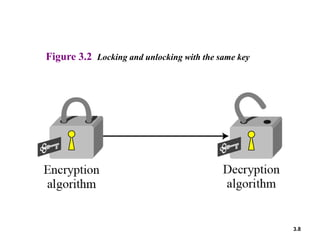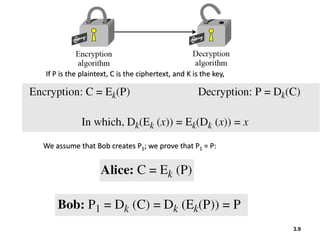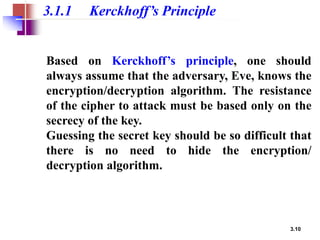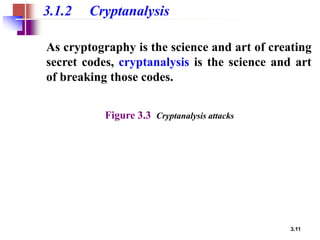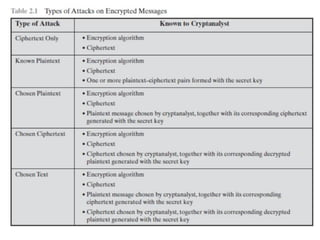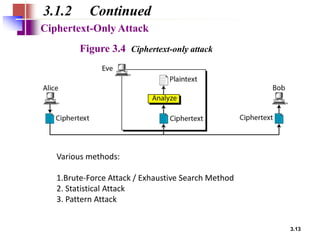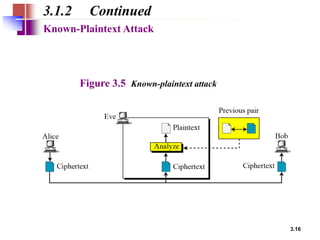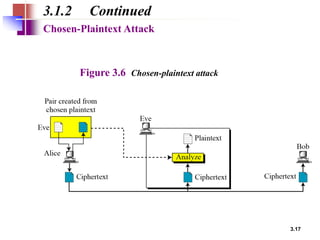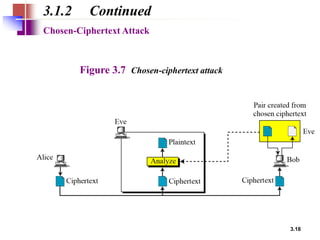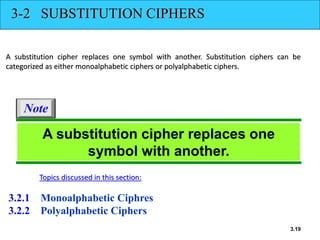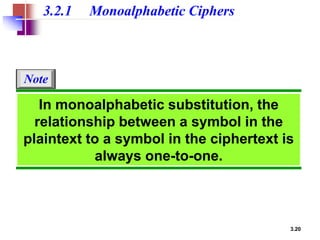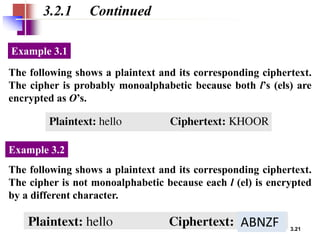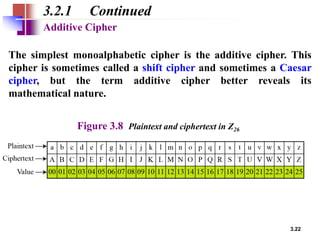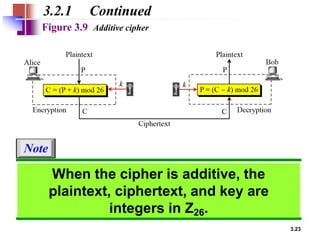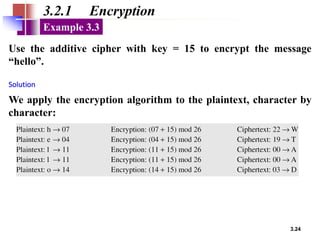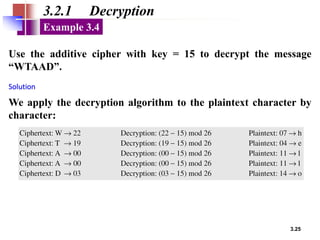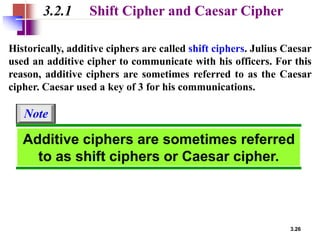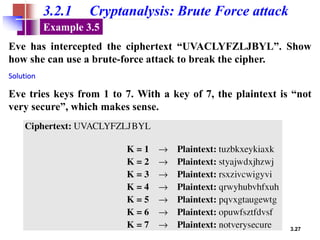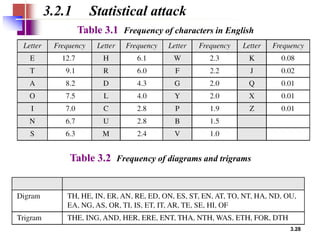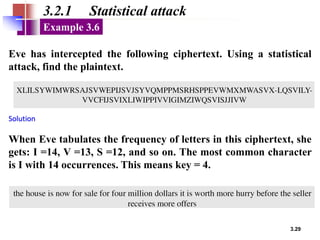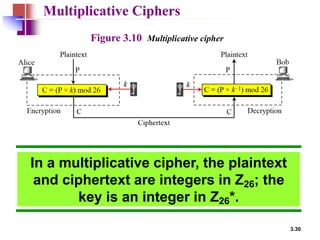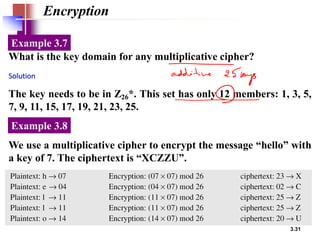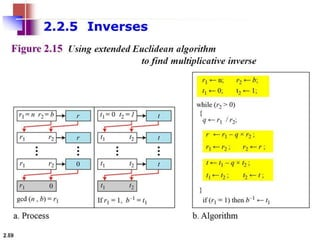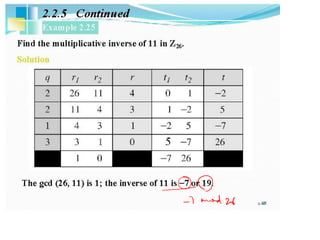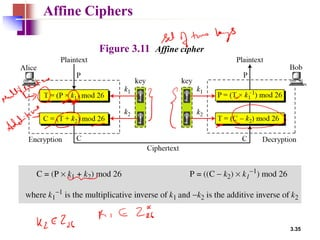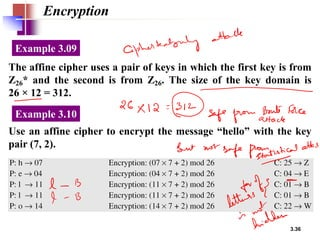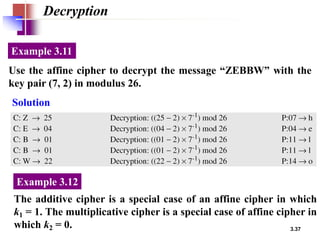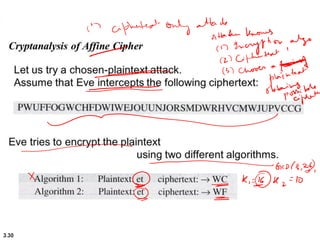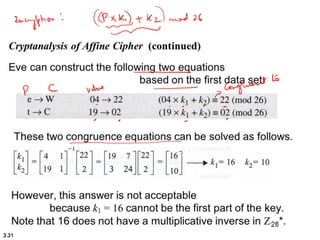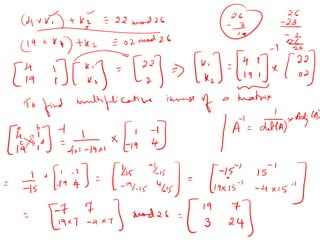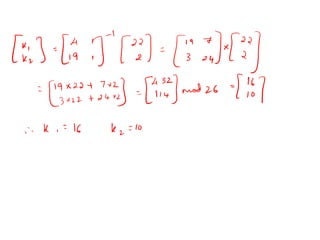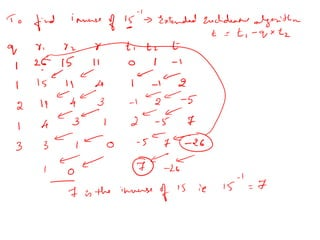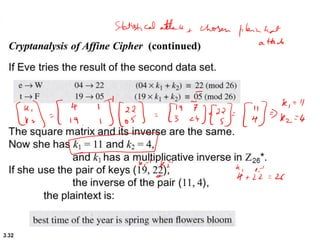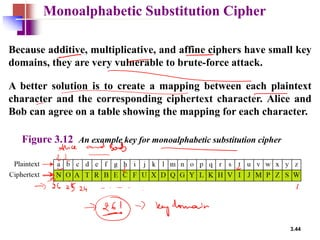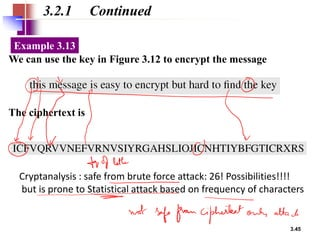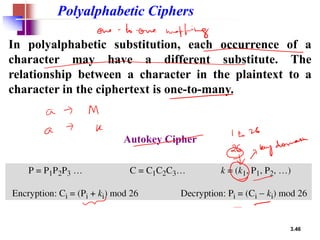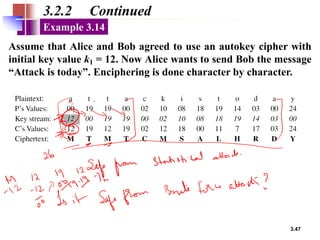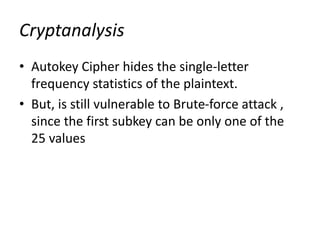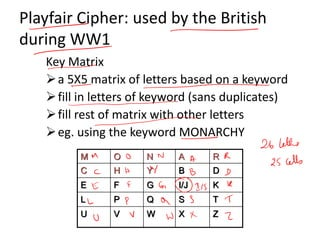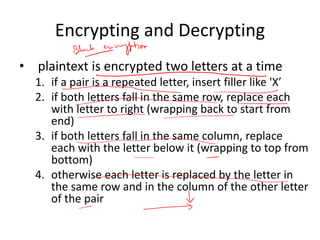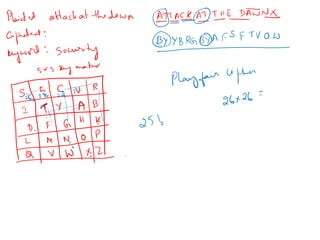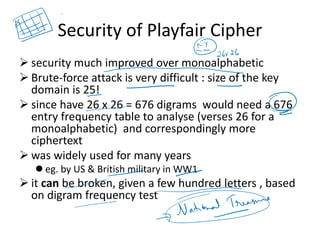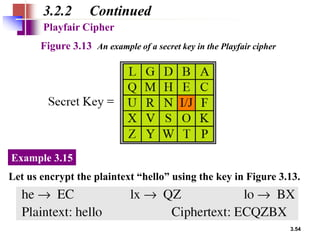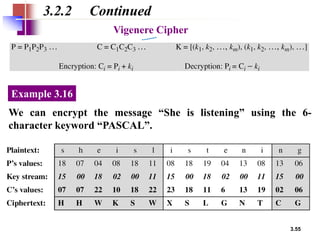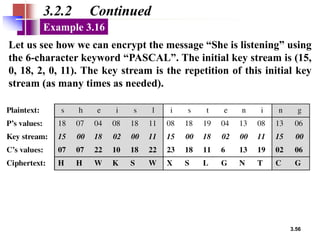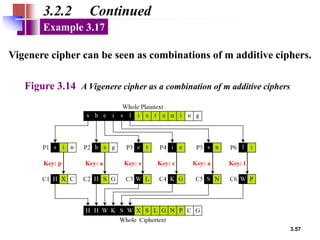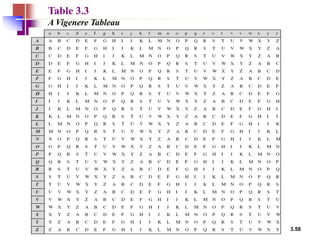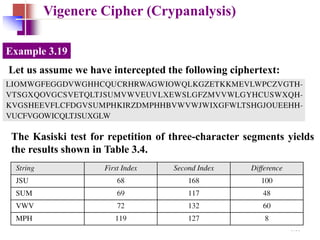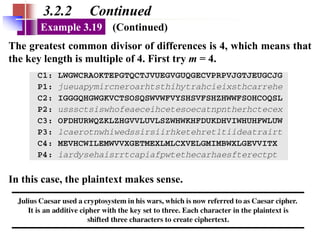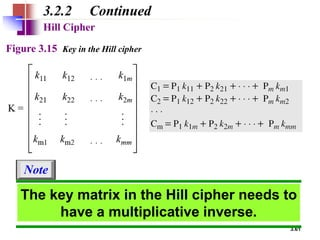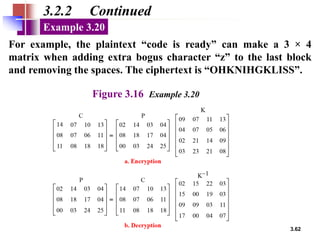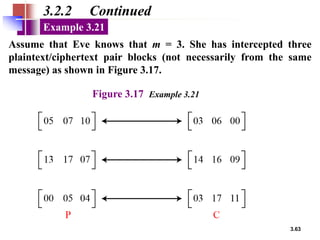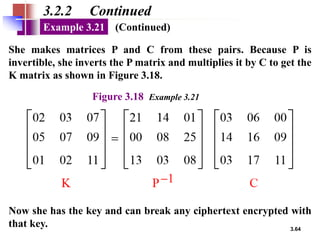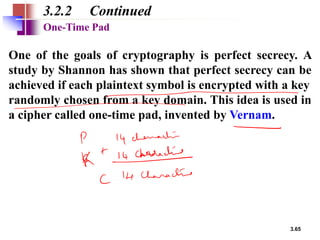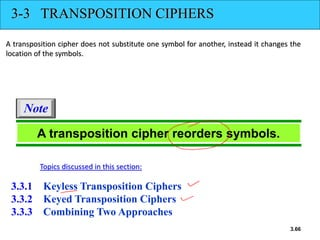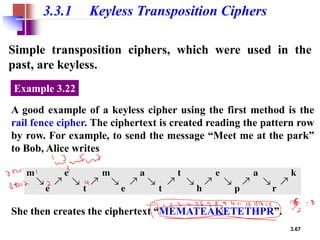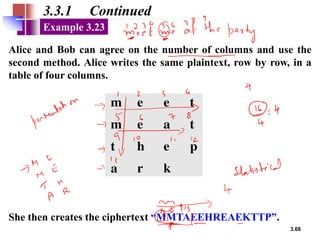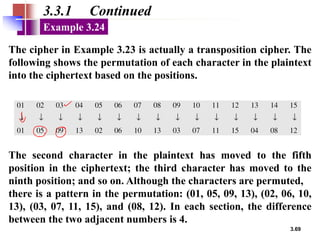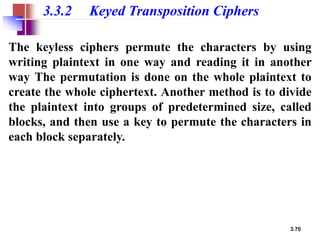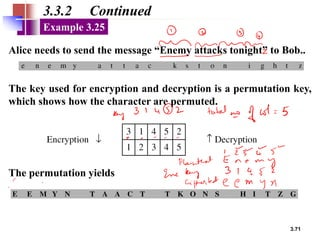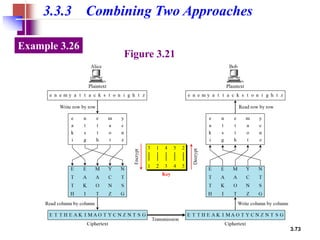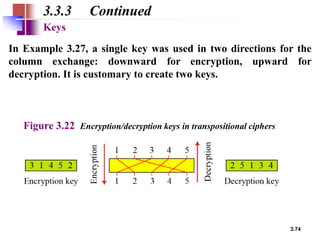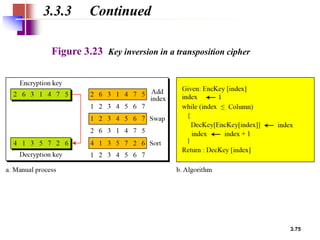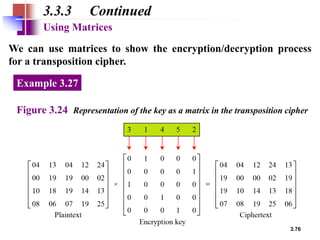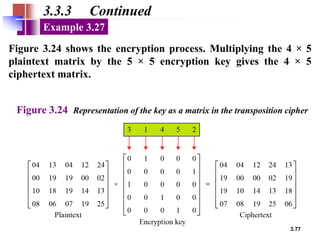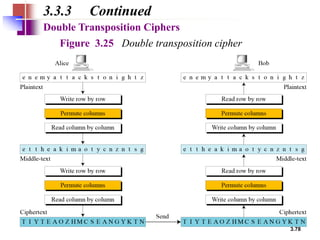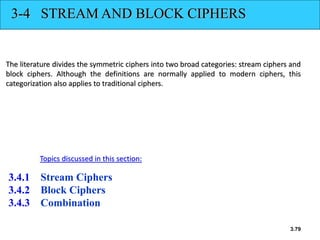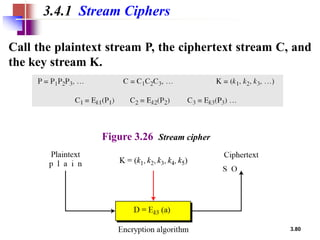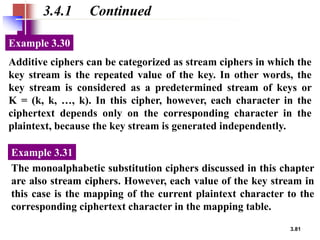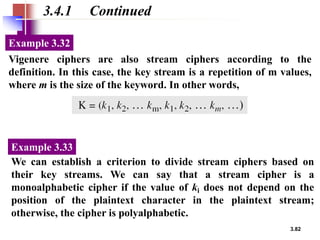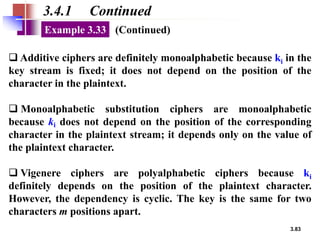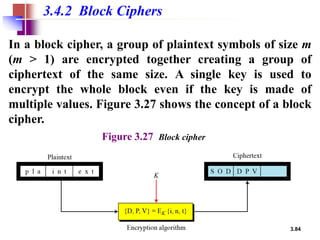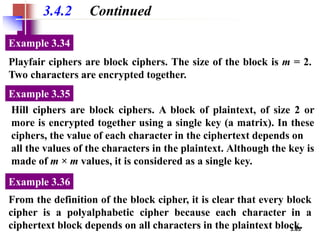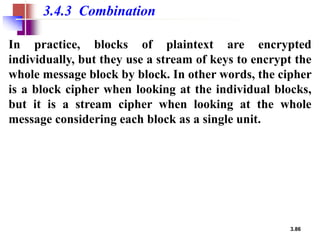1 de 87

### 2 Unit 1. Traditional Symmetric Ciphers.pdf

• 1. Traditional Symmetric key Ciphers
• 2. 3.2 ❏ To define the terms and the concepts of symmetric key ciphers ❏ To emphasize the two categories of traditional ciphers: substitution and transposition ciphers ❏ To describe the categories of cryptanalysis used to break the symmetric ciphers ❏ To introduce the concepts of the stream ciphers and block ciphers Objectives
• 3. 3.3 Figure 3.1 General idea of symmetric-key cipher 3.1 Continued
• 4. Cryptography
• 5. Basic concepts
• 6. Basic concepts
• 8. 3.8 Figure 3.2 Locking and unlocking with the same key
• 9. 3.9 If P is the plaintext, C is the ciphertext, and K is the key, We assume that Bob creates P1; we prove that P1 = P:
• 10. 3.10 3.1.1 Kerckhoff’s Principle Based on Kerckhoff’s principle, one should always assume that the adversary, Eve, knows the encryption/decryption algorithm. The resistance of the cipher to attack must be based only on the secrecy of the key. Guessing the secret key should be so difficult that there is no need to hide the encryption/ decryption algorithm.
• 11. 3.11 3.1.2 Cryptanalysis As cryptography is the science and art of creating secret codes, cryptanalysis is the science and art of breaking those codes. Figure 3.3 Cryptanalysis attacks
• 13. 3.13 3.1.2 Continued Figure 3.4 Ciphertext-only attack Ciphertext-Only Attack Various methods: 1.Brute-Force Attack / Exhaustive Search Method 2. Statistical Attack 3. Pattern Attack
• 16. 3.16 3.1.2 Continued Figure 3.5 Known-plaintext attack Known-Plaintext Attack
• 17. 3.17 3.1.2 Continued Figure 3.6 Chosen-plaintext attack Chosen-Plaintext Attack
• 18. 3.18 3.1.2 Continued Figure 3.7 Chosen-ciphertext attack Chosen-Ciphertext Attack
• 19. 3.19 3-2 SUBSTITUTION CIPHERS A substitution cipher replaces one symbol with another. Substitution ciphers can be categorized as either monoalphabetic ciphers or polyalphabetic ciphers. 3.2.1 Monoalphabetic Ciphres 3.2.2 Polyalphabetic Ciphers Topics discussed in this section: A substitution cipher replaces one symbol with another. Note
• 20. 3.20 3.2.1 Monoalphabetic Ciphers In monoalphabetic substitution, the relationship between a symbol in the plaintext to a symbol in the ciphertext is always one-to-one. Note
• 21. 3.21 3.2.1 Continued The following shows a plaintext and its corresponding ciphertext. The cipher is probably monoalphabetic because both l’s (els) are encrypted as O’s. Example 3.1 The following shows a plaintext and its corresponding ciphertext. The cipher is not monoalphabetic because each l (el) is encrypted by a different character. Example 3.2 ABNZF
• 22. 3.22 3.2.1 Continued The simplest monoalphabetic cipher is the additive cipher. This cipher is sometimes called a shift cipher and sometimes a Caesar cipher, but the term additive cipher better reveals its mathematical nature. Additive Cipher Figure 3.8 Plaintext and ciphertext in Z26
• 23. 3.23 Figure 3.9 Additive cipher 3.2.1 Continued When the cipher is additive, the plaintext, ciphertext, and key are integers in Z26. Note
• 24. 3.24 3.2.1 Encryption Use the additive cipher with key = 15 to encrypt the message “hello”. Example 3.3 We apply the encryption algorithm to the plaintext, character by character: Solution
• 25. 3.25 3.2.1 Decryption Use the additive cipher with key = 15 to decrypt the message “WTAAD”. Example 3.4 We apply the decryption algorithm to the plaintext character by character: Solution
• 26. 3.26 3.2.1 Shift Cipher and Caesar Cipher Historically, additive ciphers are called shift ciphers. Julius Caesar used an additive cipher to communicate with his officers. For this reason, additive ciphers are sometimes referred to as the Caesar cipher. Caesar used a key of 3 for his communications. Additive ciphers are sometimes referred to as shift ciphers or Caesar cipher. Note
• 27. 3.27 3.2.1 Cryptanalysis: Brute Force attack Eve has intercepted the ciphertext “UVACLYFZLJBYL”. Show how she can use a brute-force attack to break the cipher. Example 3.5 Eve tries keys from 1 to 7. With a key of 7, the plaintext is “not very secure”, which makes sense. Solution
• 28. 3.28 3.2.1 Statistical attack Table 3.1 Frequency of characters in English Table 3.2 Frequency of diagrams and trigrams
• 29. 3.29 3.2.1 Statistical attack Eve has intercepted the following ciphertext. Using a statistical attack, find the plaintext. Example 3.6 When Eve tabulates the frequency of letters in this ciphertext, she gets: I =14, V =13, S =12, and so on. The most common character is I with 14 occurrences. This means key = 4. Solution
• 30. 3.30 Multiplicative Ciphers In a multiplicative cipher, the plaintext and ciphertext are integers in Z26; the key is an integer in Z26*. Figure 3.10 Multiplicative cipher
• 31. 3.31 Encryption What is the key domain for any multiplicative cipher? Example 3.7 The key needs to be in Z26*. This set has only 12 members: 1, 3, 5, 7, 9, 11, 15, 17, 19, 21, 23, 25. Solution We use a multiplicative cipher to encrypt the message “hello” with a key of 7. The ciphertext is “XCZZU”. Example 3.8
• 35. 3.35 Affine Ciphers Figure 3.11 Affine cipher
• 36. 3.36 Encryption The affine cipher uses a pair of keys in which the first key is from Z26* and the second is from Z26. The size of the key domain is 26 × 12 = 312. Example 3.09 Use an affine cipher to encrypt the message “hello” with the key pair (7, 2). Example 3.10
• 37. 3.37 Decryption Use the affine cipher to decrypt the message “ZEBBW” with the key pair (7, 2) in modulus 26. Example 3.11 Solution The additive cipher is a special case of an affine cipher in which k1 = 1. The multiplicative cipher is a special case of affine cipher in which k2 = 0. Example 3.12
• 44. 3.44 Monoalphabetic Substitution Cipher Because additive, multiplicative, and affine ciphers have small key domains, they are very vulnerable to brute-force attack. A better solution is to create a mapping between each plaintext character and the corresponding ciphertext character. Alice and Bob can agree on a table showing the mapping for each character. Figure 3.12 An example key for monoalphabetic substitution cipher
• 45. 3.45 3.2.1 Continued We can use the key in Figure 3.12 to encrypt the message Example 3.13 The ciphertext is Cryptanalysis : safe from brute force attack: 26! Possibilities!!!! but is prone to Statistical attack based on frequency of characters
• 46. 3.46 Polyalphabetic Ciphers In polyalphabetic substitution, each occurrence of a character may have a different substitute. The relationship between a character in the plaintext to a character in the ciphertext is one-to-many. Autokey Cipher
• 47. 3.47 3.2.2 Continued Assume that Alice and Bob agreed to use an autokey cipher with initial key value k1 = 12. Now Alice wants to send Bob the message “Attack is today”. Enciphering is done character by character. Example 3.14
• 48. Cryptanalysis • Autokey Cipher hides the single-letter frequency statistics of the plaintext. • But, is still vulnerable to Brute-force attack , since the first subkey can be only one of the 25 values
• 49. Playfair Cipher: used by the British during WW1 Key Matrix ➢a 5X5 matrix of letters based on a keyword ➢fill in letters of keyword (sans duplicates) ➢fill rest of matrix with other letters ➢eg. using the keyword MONARCHY M O N A R C H Y B D E F G I/J K L P Q S T U V W X Z
• 50. Encrypting and Decrypting • plaintext is encrypted two letters at a time 1. if a pair is a repeated letter, insert filler like 'X’ 2. if both letters fall in the same row, replace each with letter to right (wrapping back to start from end) 3. if both letters fall in the same column, replace each with the letter below it (wrapping to top from bottom) 4. otherwise each letter is replaced by the letter in the same row and in the column of the other letter of the pair
• 53. Security of Playfair Cipher ➢ security much improved over monoalphabetic ➢ Brute-force attack is very difficult : size of the key domain is 25! ➢ since have 26 x 26 = 676 digrams would need a 676 entry frequency table to analyse (verses 26 for a monoalphabetic) and correspondingly more ciphertext ➢ was widely used for many years ⚫ eg. by US & British military in WW1 ➢ it can be broken, given a few hundred letters , based on digram frequency test
• 54. 3.54 3.2.2 Continued Playfair Cipher Figure 3.13 An example of a secret key in the Playfair cipher Let us encrypt the plaintext “hello” using the key in Figure 3.13. Example 3.15
• 55. 3.55 3.2.2 Continued Vigenere Cipher We can encrypt the message “She is listening” using the 6- character keyword “PASCAL”. Example 3.16
• 56. 3.56 3.2.2 Continued Let us see how we can encrypt the message “She is listening” using the 6-character keyword “PASCAL”. The initial key stream is (15, 0, 18, 2, 0, 11). The key stream is the repetition of this initial key stream (as many times as needed). Example 3.16
• 57. 3.57 3.2.2 Continued Vigenere cipher can be seen as combinations of m additive ciphers. Example 3.17 Figure 3.14 A Vigenere cipher as a combination of m additive ciphers
• 58. 3.58 Table 3.3 A Vigenere Tableau
• 59. 3.59 Vigenere Cipher (Crypanalysis) Let us assume we have intercepted the following ciphertext: Example 3.19 The Kasiski test for repetition of three-character segments yields the results shown in Table 3.4.
• 60. 3.60 3.2.2 Continued The greatest common divisor of differences is 4, which means that the key length is multiple of 4. First try m = 4. Example 3.19 (Continued) In this case, the plaintext makes sense.
• 61. 3.61 3.2.2 Continued Hill Cipher Figure 3.15 Key in the Hill cipher The key matrix in the Hill cipher needs to have a multiplicative inverse. Note
• 62. 3.62 3.2.2 Continued For example, the plaintext “code is ready” can make a 3 × 4 matrix when adding extra bogus character “z” to the last block and removing the spaces. The ciphertext is “OHKNIHGKLISS”. Example 3.20 Figure 3.16 Example 3.20
• 63. 3.63 3.2.2 Continued Assume that Eve knows that m = 3. She has intercepted three plaintext/ciphertext pair blocks (not necessarily from the same message) as shown in Figure 3.17. Example 3.21 Figure 3.17 Example 3.21
• 64. 3.64 3.2.2 Continued She makes matrices P and C from these pairs. Because P is invertible, she inverts the P matrix and multiplies it by C to get the K matrix as shown in Figure 3.18. Example 3.21 Figure 3.18 Example 3.21 Now she has the key and can break any ciphertext encrypted with that key. (Continued)
• 65. 3.65 3.2.2 Continued One of the goals of cryptography is perfect secrecy. A study by Shannon has shown that perfect secrecy can be achieved if each plaintext symbol is encrypted with a key randomly chosen from a key domain. This idea is used in a cipher called one-time pad, invented by Vernam. One-Time Pad
• 66. 3.66 3-3 TRANSPOSITION CIPHERS A transposition cipher does not substitute one symbol for another, instead it changes the location of the symbols. 3.3.1 Keyless Transposition Ciphers 3.3.2 Keyed Transposition Ciphers 3.3.3 Combining Two Approaches Topics discussed in this section: A transposition cipher reorders symbols. Note
• 67. 3.67 3.3.1 Keyless Transposition Ciphers Simple transposition ciphers, which were used in the past, are keyless. A good example of a keyless cipher using the first method is the rail fence cipher. The ciphertext is created reading the pattern row by row. For example, to send the message “Meet me at the park” to Bob, Alice writes Example 3.22 She then creates the ciphertext “MEMATEAKETETHPR”.
• 68. 3.68 3.3.1 Continued Alice and Bob can agree on the number of columns and use the second method. Alice writes the same plaintext, row by row, in a table of four columns. Example 3.23 She then creates the ciphertext “MMTAEEHREAEKTTP”.
• 69. 3.69 3.3.1 Continued The cipher in Example 3.23 is actually a transposition cipher. The following shows the permutation of each character in the plaintext into the ciphertext based on the positions. Example 3.24 The second character in the plaintext has moved to the fifth position in the ciphertext; the third character has moved to the ninth position; and so on. Although the characters are permuted, there is a pattern in the permutation: (01, 05, 09, 13), (02, 06, 10, 13), (03, 07, 11, 15), and (08, 12). In each section, the difference between the two adjacent numbers is 4.
• 70. 3.70 3.3.2 Keyed Transposition Ciphers The keyless ciphers permute the characters by using writing plaintext in one way and reading it in another way The permutation is done on the whole plaintext to create the whole ciphertext. Another method is to divide the plaintext into groups of predetermined size, called blocks, and then use a key to permute the characters in each block separately.
• 71. 3.71 3.3.2 Continued Alice needs to send the message “Enemy attacks tonight” to Bob.. Example 3.25 The key used for encryption and decryption is a permutation key, which shows how the character are permuted. The permutation yields
• 73. 3.73 3.3.3 Combining Two Approaches Example 3.26 Figure 3.21
• 74. 3.74 Figure 3.22 Encryption/decryption keys in transpositional ciphers 3.3.3 Continued Keys In Example 3.27, a single key was used in two directions for the column exchange: downward for encryption, upward for decryption. It is customary to create two keys.
• 75. 3.75 Figure 3.23 Key inversion in a transposition cipher 3.3.3 Continued
• 76. 3.76 3.3.3 Continued Using Matrices We can use matrices to show the encryption/decryption process for a transposition cipher. Figure 3.24 Representation of the key as a matrix in the transposition cipher Example 3.27
• 77. 3.77 Figure 3.24 Representation of the key as a matrix in the transposition cipher 3.3.3 Continued Figure 3.24 shows the encryption process. Multiplying the 4 × 5 plaintext matrix by the 5 × 5 encryption key gives the 4 × 5 ciphertext matrix. Example 3.27
• 78. 3.78 3.3.3 Continued Double Transposition Ciphers Figure 3.25 Double transposition cipher
• 79. 3.79 3-4 STREAM AND BLOCK CIPHERS The literature divides the symmetric ciphers into two broad categories: stream ciphers and block ciphers. Although the definitions are normally applied to modern ciphers, this categorization also applies to traditional ciphers. 3.4.1 Stream Ciphers 3.4.2 Block Ciphers 3.4.3 Combination Topics discussed in this section:
• 80. 3.80 3.4.1 Stream Ciphers Call the plaintext stream P, the ciphertext stream C, and the key stream K. Figure 3.26 Stream cipher
• 81. 3.81 3.4.1 Continued Additive ciphers can be categorized as stream ciphers in which the key stream is the repeated value of the key. In other words, the key stream is considered as a predetermined stream of keys or K = (k, k, …, k). In this cipher, however, each character in the ciphertext depends only on the corresponding character in the plaintext, because the key stream is generated independently. Example 3.30 The monoalphabetic substitution ciphers discussed in this chapter are also stream ciphers. However, each value of the key stream in this case is the mapping of the current plaintext character to the corresponding ciphertext character in the mapping table. Example 3.31
• 82. 3.82 3.4.1 Continued Vigenere ciphers are also stream ciphers according to the definition. In this case, the key stream is a repetition of m values, where m is the size of the keyword. In other words, Example 3.32 We can establish a criterion to divide stream ciphers based on their key streams. We can say that a stream cipher is a monoalphabetic cipher if the value of ki does not depend on the position of the plaintext character in the plaintext stream; otherwise, the cipher is polyalphabetic. Example 3.33
• 83. 3.83 3.4.1 Continued ❑ Additive ciphers are definitely monoalphabetic because ki in the key stream is fixed; it does not depend on the position of the character in the plaintext. ❑ Monoalphabetic substitution ciphers are monoalphabetic because ki does not depend on the position of the corresponding character in the plaintext stream; it depends only on the value of the plaintext character. ❑ Vigenere ciphers are polyalphabetic ciphers because ki definitely depends on the position of the plaintext character. However, the dependency is cyclic. The key is the same for two characters m positions apart. Example 3.33 (Continued)
• 84. 3.84 3.4.2 Block Ciphers In a block cipher, a group of plaintext symbols of size m (m > 1) are encrypted together creating a group of ciphertext of the same size. A single key is used to encrypt the whole block even if the key is made of multiple values. Figure 3.27 shows the concept of a block cipher. Figure 3.27 Block cipher
• 85. 3.85 3.4.2 Continued Playfair ciphers are block ciphers. The size of the block is m = 2. Two characters are encrypted together. Example 3.34 Hill ciphers are block ciphers. A block of plaintext, of size 2 or more is encrypted together using a single key (a matrix). In these ciphers, the value of each character in the ciphertext depends on all the values of the characters in the plaintext. Although the key is made of m × m values, it is considered as a single key. Example 3.35 From the definition of the block cipher, it is clear that every block cipher is a polyalphabetic cipher because each character in a ciphertext block depends on all characters in the plaintext block. Example 3.36
• 86. 3.86 3.4.3 Combination In practice, blocks of plaintext are encrypted individually, but they use a stream of keys to encrypt the whole message block by block. In other words, the cipher is a block cipher when looking at the individual blocks, but it is a stream cipher when looking at the whole message considering each block as a single unit.